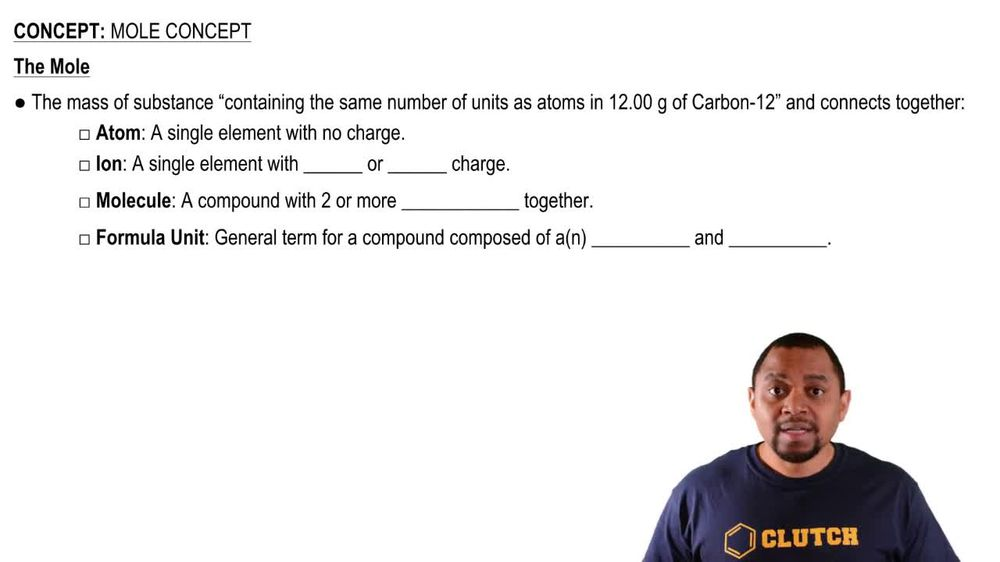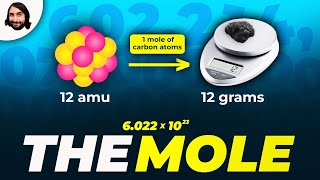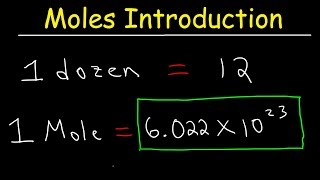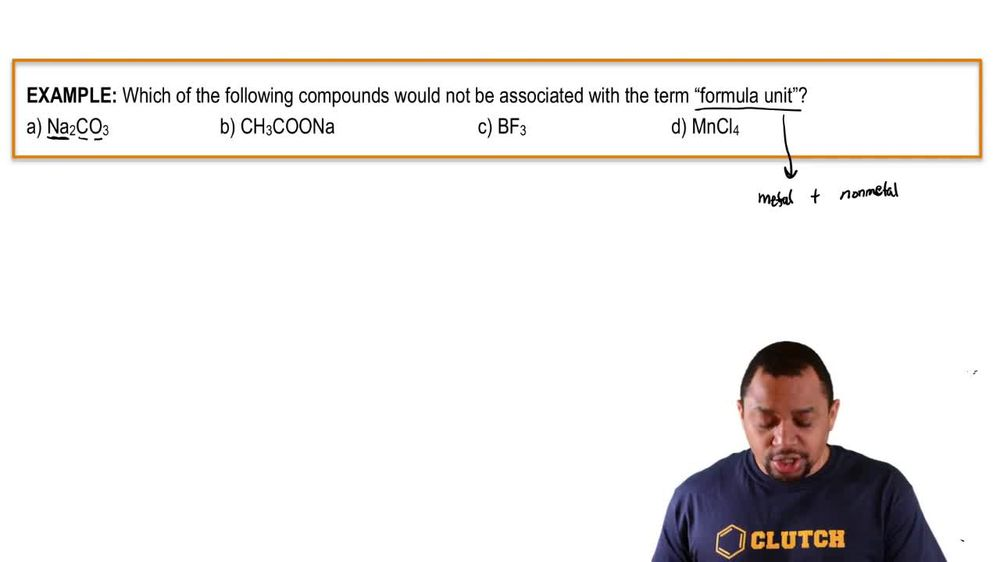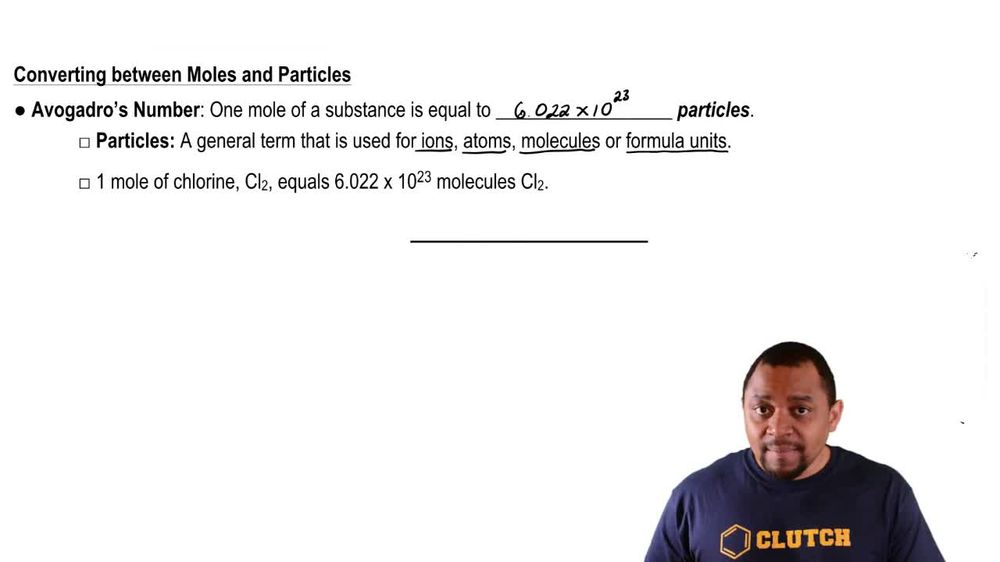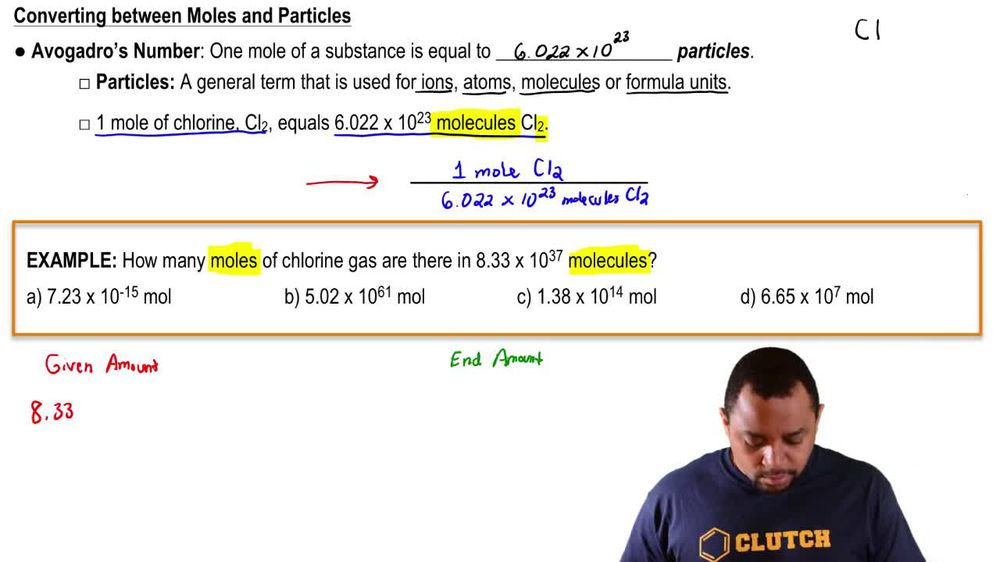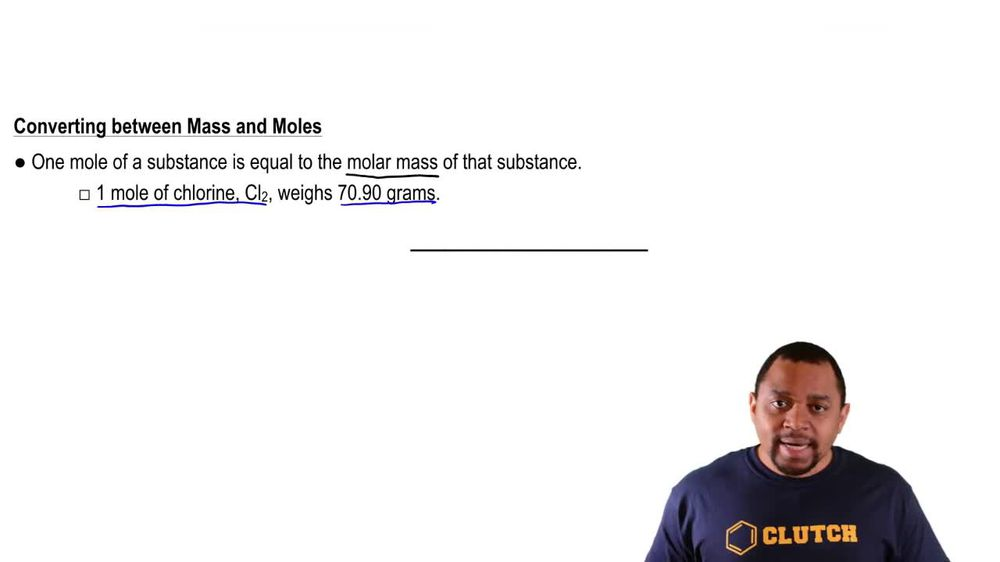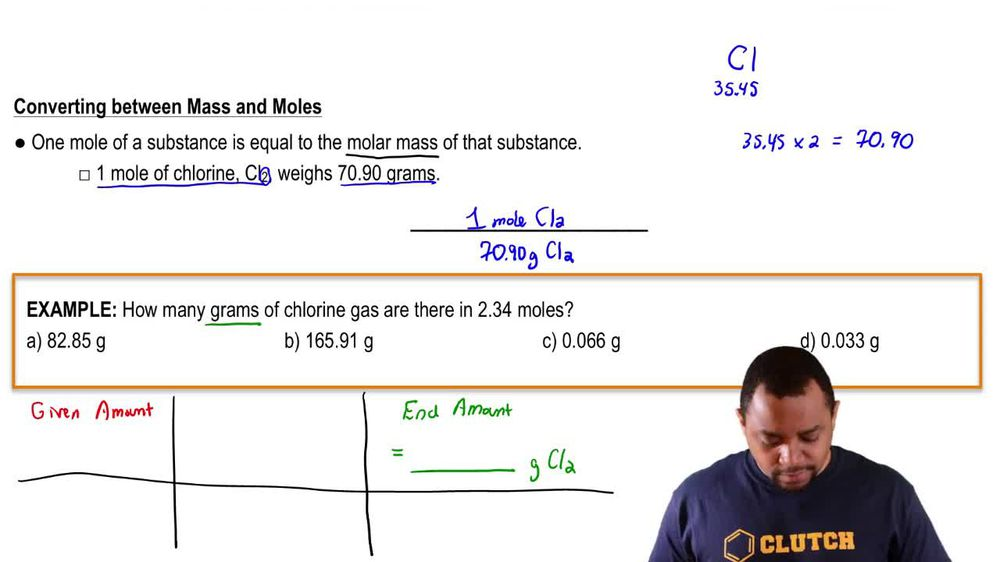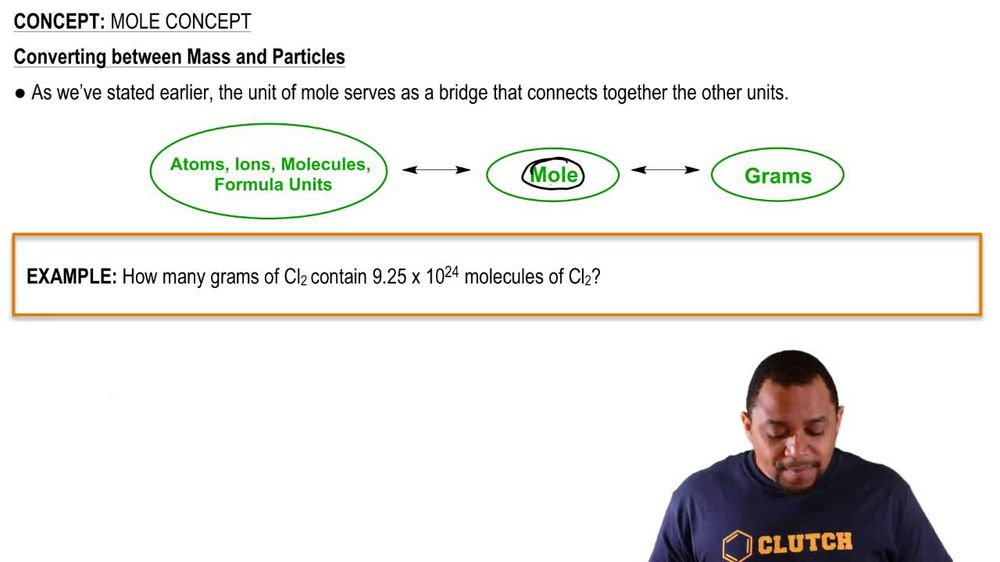Start typing, then use the up and down arrows to select an option from the list.
1. 2. Atoms & Elements2. Mole Concept
Problem

# Iron(II) sulfate, FeSO4, is prescribed for the treatment of anemia. How many moles of FeSO4 are present in a standard 300 mg tablet? How many iron(II) ions?

Relevant Solution2m
Play a video:
Hey everyone welcome back. So let's get started with this video. So here they want us to calculate the number of B r minus ions and 56.87 g of MGB R two. Okay, so here we are given 56.87 g. So we're starting off with g and then we're going to want to go to malls. So how do we go from grams to moles? We're going to use the molar mass of MGB R two And we find that to be 184 point 11 g per mole. Okay, and then for moles we're going to find the most of B R minus NGR bureau to contains. Then finally we're going to go to ions which is what we're looking for. And how do we go for most of the r minus two ions? We're going to use avocados number Which is 6.02. 2 times 10 to the 23. Okay, so with that being said, let's go ahead and start our store Akiyama Tree. So they start with the given which is 56 point 87 g of MG B R two. Okay, so we're going to use a molar mass to go from grams to moles. So then there is 184 .1, 1 grounds of MG B R two and one more of MG B R two. Okay then for most we're going to find the most will be our minus by looking at the formula. So then one more of MG B R two contains to be our minus ions. Two malls of P R minus and then finally what we're going to do and use avocados numbers to solve for ions. So then one mole of r minus is 6.0-2 Times 10 to the 23 P r minus ions. So then grams of MGP are to cancel out most of em gpr to cancel out most of p r minus, cancel out. We do the math and we get three 0. times 10 To the B r minus ions. Okay, thank you for watching. I hope this helped and I'll see you in the next video.